#Nonhomogeneous Second Order Linear EquationsLet us go back to the nonhomogeneous second order linear equations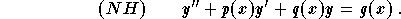Recall that the general solution is given by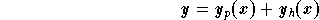where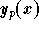is a particular solution of (NH) and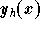is the general solution of the associated homogeneous equation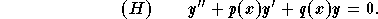In the previous sections we discussed how to find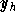. In this section we will discuss two major techniques giving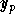:[Differential Equations] [First Order D.E.] [Second Order D.E.]
[Geometry] [Algebra] [Trigonometry ]
[Calculus] [Complex Variables] [Matrix Algebra]S.O.S MATHematics home page

Do you need more help? Please post your question on our S.O.S. Mathematics CyberBoard.Author: Mohamed Amine Khamsi